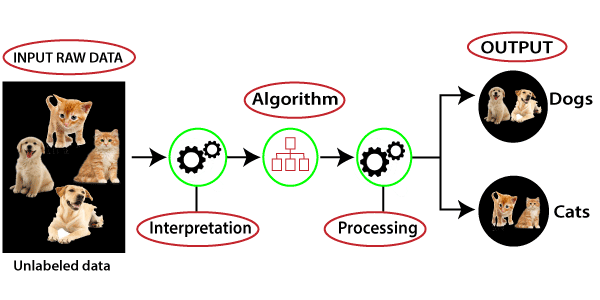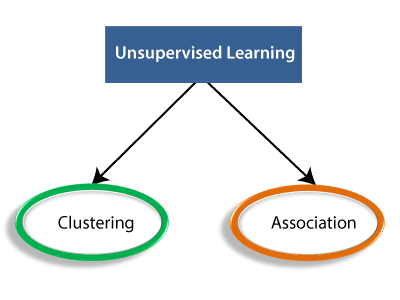# How Unsupervised learning works?

## Working of Unsupervised Learning

Working of unsupervised learning can be understood by the below diagram:Here, we have taken an unlabeled input data, which means it is not categorized and corresponding outputs are also not given. Now, this unlabeled input data is fed to the machine learning model in order to train it. Firstly, it will interpret the raw data to find the hidden patterns from the data and then will apply suitable algorithms such as k-means clustering, Decision tree, etc.

Once it applies the suitable algorithm, the algorithm divides the data objects into groups according to the similarities and difference between the objects.

## Types of Unsupervised Learning Algorithm:

The unsupervised learning algorithm can be further categorized into two types of problems:• Clustering : Clustering is a method of grouping the objects into clusters such that objects with most similarities remains into a group and has less or no similarities with the objects of another group. Cluster analysis finds the commonalities between the data objects and categorizes them as per the presence and absence of those commonalities.
• Association : An association rule is an unsupervised learning method which is used for finding the relationships between variables in the large database. It determines the set of items that occurs together in the dataset. Association rule makes marketing strategy more effective. Such as people who buy X item (suppose a bread) are also tend to purchase Y (Butter/Jam) item. A typical example of Association rule is Market Basket Analysis.

## Unsupervised Learning algorithms:

Below is the list of some popular unsupervised learning algorithms:

• K-means clustering
• KNN (k-nearest neighbors)
• Hierarchal clustering
• Anomaly detection
• Neural Networks
• Principle Component Analysis
• Independent Component Analysis
• Apriori algorithm
• Singular value decomposition